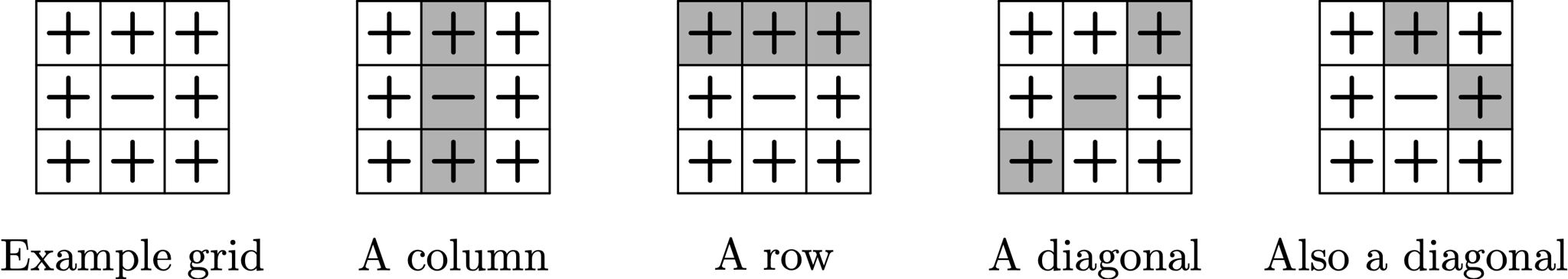시간 제한 메모리 제한 제출 정답 맞은 사람 정답 비율
2 초 256 MB 3 1 1 33.333%

## 문제

You are given a $n \times m$ table, with each cell containing a + or a -. You are allowed to flip any row, column or diagonal of the table:When you flip a set of cells, all +s are changed to -s and vice versa. Note that there are $n$ rows, $m$ columns, and $2(n+m-1)$ diagonals in a $n \times m$ grid. Some diagonals consist of just one cell.

Can you change the given table into a table full of +s?

## 입력

The first line of the input file contains two integers $n$ and $m$ --- the dimensions of the table ($1 \le n, m \le 1000$). The next $n$ lines contain $m$ characters each, describing the table. Each of those characters is either + or -.

## 출력

If it's possible to change the given table into a table with only +s, print Ja on the first line of the output file, otherwise print Nein. In case you printed Ja, on the second line print the number $k$ of flips that you're going to use. On the following $k$ lines print the descriptions of flips, one per line. Each flip is given by the coordinates of any two different cells it involves. In case there's just one cell in the row, column or diagonal being flipped, and only in that case, repeat the same cell twice. The first coordinate of each cell is between 1 and $n$, and the second is between 1 and $m$. The cell with coordinates 1 1 corresponds to the first character of the first line of the input table.

Note that you don't need to minimize the number of moves $k$. The only constraint is that it must not exceed 10000.

## 예제 입력 1

5 6
------
------
------
------
------


## 예제 출력 1

Ja
6
1 1 5 1
1 2 5 2
1 3 5 3
1 4 5 4
1 5 5 5
1 6 5 6


## 예제 입력 2

3 3
+++
+-+
+++


## 예제 출력 2

Ja
3
1 2 3 2
2 3 3 2
1 2 2 3